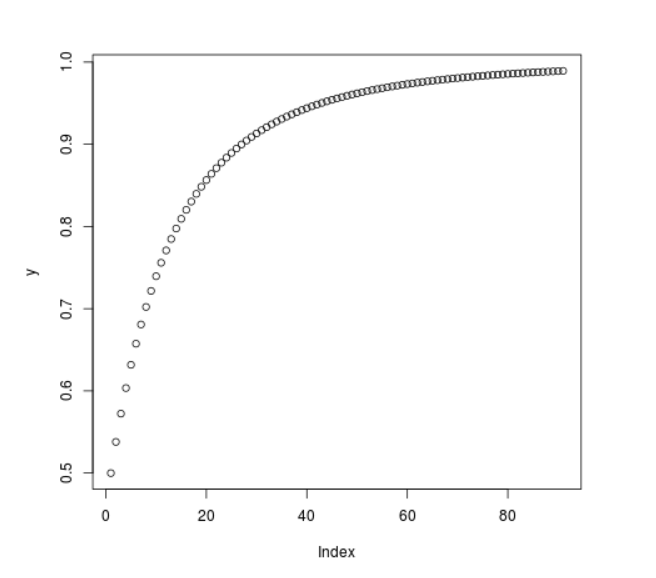Open in App
Not now

# Compute Cumulative Log Normal Probability Density in R Programming – plnorm() Function

• Last Updated : 25 Jun, 2020

`plnorm()` function in R Language is used to compute the log normal value of the cumulative probability density function. It also creates a plot of the cumulative distribution of log normal density.

Syntax: plnorm(vec)

Parameters:
vec: x-values for normal density

Example 1:

 `# R program to compute cumulative``# log normal probability density`` ` `# Creating x-values for density``x <``-` `seq(``1``, ``10``, by ``=` `1``)`` ` `# Calling plnorm() function``y <``-` `plnorm(x)``y`

Output:

```  0.5000000 0.7558914 0.8640314 0.9171715 0.9462397 0.9634142 0.9741672
 0.9812116 0.9859978 0.9893489
```

Example 2:

 `# R program to compute cumulative``# log normal probability density`` ` `# Creating x-values for density``x <``-` `seq(``1``, ``10``, by ``=` `0.1``)`` ` `# Calling plnorm() function``y <``-` `plnorm(x)`` ` `# Plot a graph``plot(y)`

Output:My Personal Notes arrow_drop_up